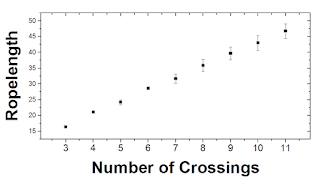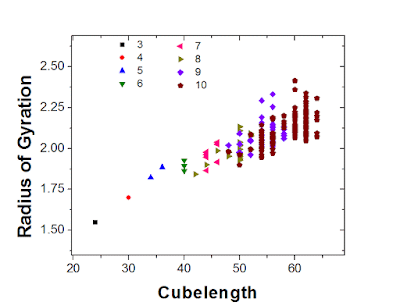## Thursday, 3 November 2016

### DNA, topological ropelength, and minimal lattice knots

This post is about some diversions into knot theory, a branch of topology, that I took while contemplating my experiments. My job involves performing and analyzing experiments where I stretch out DNA molecules with knots in them. We study DNA because it serves as a good model polymer that's analogous to the much smaller polymer molecules that plastics and other modern materials are made of, and we study knots because we want to understand how entanglement affects polymer dynamics. There are also some genetic sequencing technologies for which we might want to add or remove knots, but right now I'm mainly just trying to figure out how polymer knots work. The theoretical side of the project (largely carried out by my colleague Vivek and Liang) involves running simulations of polymer chains with different kinds of knots, or simulating the formation of knots, and thinking about that is where I start to dip into knot theory.A DNA molecule with two knots in it, from one of my experiments. The molecule is about 50 microns when stretched.

Mathematically, knots are only truly defined in loops, as a knot in an open string can just be slid off the end. Knots are loosely categorized by the minimum number of times the loop crosses over itself in a diagram (see below). The simplest knot, the trefoil, when drawn must cross itself a minimum of three times. For a given crossing number, there can be multiple kinds of knots. The two simplest knots, with three and four crossings, only have one type, then there are two for five, three for six, seven for seven, then it starts to explode:  165 for ten crossings, 9988 for thirteen, and over a million for sixteen. Beyond this, it is unknown how many kinds of knots there are (although there are constraints). Each type of knot has certain parameters that are unique to the  knot, called invariants, and calculating these invariants is how knots can be distinguished. When dealing with knots in real ropes and polymers and DNA, we have to remember that it doesn't count as a knot unless the ends are closed. If the ends are very far from the interesting knotty part we can just pretend this is the case without too much issue; in simulations of tightly bunched knots however there are various algorithms called "minimally interfering closure" that make knots in open strings topologically kosher.Knots with up to seven crossings.
The typical model of a polymer is that of a self-avoiding walk, which I've talked about in the past. When the walk is forced to have closed ends, it represents a topologically circular molecule, and can be thought of as a self avoiding polygon (even though polygons are typically imagined in two dimensions). These self-avoiding polygons can take the form of different types of knots, and in fact it can be shown that as a self-avoiding walk becomes longer and longer, the probability of having a knot in it approaches 100%. A number of computational papers have shown that knotted ring polymers have a size (quantified by the radius of gyration, the standard deviation of the location of each monomer in the chain) which is smaller than an unknotted chain of the same length.  This makes intuitive sense, there are topological constraints which prevent the chain from adopting a widespread conformation.

The chains in these simulations tend to be quite "loose," and I was curious about the scaling of tight or  "ideal" knots, which are knots that have the minimum possible length-to-diameter ratio. Each type of knot has a parameter called the ropelength, which is the minimum length required to make a knot of unit diameter. The ropelength can be a difficult quantity to calculate, and one group has written a few papers just slightly hammering down the ropelength of the simplest knot, bringing it from 16.38 in 2001 to 16.37 in 2014.These knots are "ideal" meaning they have the smallest possible length-diameter ratio.

The ropelength comes up in my research in part because of a theory from Grosberg and Rabin, who derived the free energy of a knot in a polymer. The free energy arises from the fact that the length of polymer in the knot is confined, reducing entropy, and bent, increasing energy. They argued that the energy of a given knot type is minimized at a certain length, and such a knotted conformation constitutes a metastable local minimum in the free energy (compared to the unknotted global minimum) that implies that an open knotted polymer wouldn't spontaneously unknot itself, but instead would unknot through a slower diffusion process when the knot reaches one end. One of the terms in the Grosberg-Rabin is the excess knot contour, which is the length of polymer in the knot minus the ropelength, and thus it is necessary to know the ropelength to calculate the metastable knot energy. Another paper argued that metastable knots don't exist, and that is one of the things I'm trying to figure out with my knotted DNA experiments.

The most topologically accurate DNA knot experiment comes from Robert Bao, Heun Jin Lee, and Stephen Quake in 2003, where knots of known topology were tied in individual DNA molecules using optical tweezers. I get the impression that Bao developed the expertise to do this, wrote his paper, and made the sanity-promoting decision never to do it again. In their paper, they measured the amount of DNA in different kinds of knots and the diffusion of the knots along the molecule, and used this to ascertain how much intramolecular friction was occurring within the knot.  Grosberg and Rabin claimed to have postdicted Bao et al.'s findings with their model. In my experiments, unfortunately, the knots are much more complicated and I don't know what kind they are.

In my experiments, I'm interested in figuring out what kind of knots I'm looking at, and one way to do that is to estimate the amount of DNA in the knot and backstrapolating to figure out the number of essential crossings. To aid in this endeavor, I looked up the known ropelength of knots up to 11 crossings, which was not super-easy nor super-difficult to find. I don't think anyone has analyzed this specific relationship before, but not too surprisingly, with more crossings you tend to have a longer knot, although there is overlap between knots with adjacent or superadjacent crossing number. This relationship fits well with either a linear or square-root function (the best-fit power is 0.8...not sure that's meaningful but I love power-law fits). Once you get past 11 crossings there are problems because A. nobody has bothered calculating this for 12-crossing knots (of which there are 2176) and B. the mean isn't a useful parameter anymore because there are two populations: alternating and non-alternating knots, which have their own behaviours. Complex knots often have more in common with other knots of a certain class (e.g. twist, torus knots) than with the same crossing number.More complex knots require more rope to tie.
My idea was to use this trend to figure out or constrain the topology in my experiments. For example, if my knot has 2 microns of DNA in it, I can figure out (hypothetically) that it must have at most 30 crossings based on extrapolating the ropelength curve or Bao's data, or something like that. I still haven't solved the topology-constraining problem. However, looking into the ropelength scaling got me interested in other aspects of maximally tight knots, even though maximally tight knots don't really occur in my experiments.

In addition to the lengths of ideal knots, I was interested in their size in general, which for a polymer is typically quantified by the radius of gyration, and a lot of polymer physics looks at the scaling of the radius of gyration with length. It's established that loose floppy knots grow more slowly than loose floppy rings, but what about tight knots? It turns out, the radii of gyration for ideal knots hasn't been calculated (at least not to my knowledge), so to examine this I'd have to generate the knots based on tables of 100 Fourier coefficients and then discretize and measure them. Instead I opted to look at a less-ideal system, minimal knots on cubic lattices. These were tabulated by Andrew Rechnitzer for a paper on the topic, and exist for knots up to 10 crossings (for which there are 165). These are useful for doing simulations of knotted polymers because they allow the initialization of complex knots, and the lack of tabulation for more complex knots makes them difficult to systematically simulate.A minimal 10-crossing knot on a cubic lattice.
These are the knots whose coordinates on a cubic lattice have the least number of points, but they are still longer than ideal knots. How much longer? The length of each minimal lattice knot (which I call the cubelength) is roughly 40% greater than the equivalent ideal knot, decreasing slightly as a function of crossing number. This makes handwaving sense, because you have an extra factor of square root of two if you go across a diagonal instead of taking two steps. We can see all the different knots (from 3 to 10 crossings) in the graph below; notice there is overlap between the largest 7-crossing knots and the smallest 10-crossing knots. I had a hypothesis that these tight knotted lattice polygons would be more compact in their scaling (the radius of gyration would increase with a weaker power of length) than regular compact lattice walks, and went to examine it.Every minimal lattice knot up to 10 crossings (except one of the 10-knots...I only ended up with 164 for some reason).

The size of a random walk grows with the square root or 0.5 power of its length. The size of a self-avoiding walk grows faster, with roughly the 0.6 power, for reasons I explain here. A compact globule (the form a polymer takes when it collapses) which grows by wrapping around itself, will grow with a volume proportional to its length, so its radius is expected to grow with the 0.33 power of its length. What do we see when we look at lattice knots? The ensemble of compact lattice knots grows at the 0.33+-0.02 power, entirely consistent with a compact globule.Left: Average izes of minimal cubic lattice knots as a function of the crossing number. Right, same as a function of their length. I intuitively guessed the function Rg=(L/6)$^{1/3}$ and it fit the data really well.
Nothing too exciting there, but another question one could ask is whether minimal knots grow faster or slower than random self-attracted walks of comparable length, as polymers scale differently as they grow, before they reach the asymptotic limit. To figure this out, I generated self-avoiding random walks on a cubic lattice up to length 60, biasing the steps they take (with a Metropolis method) to have a strong preference for being next to non-adjacent occupied sites. Even with very strong self-attraction (well past the theta-point in polymer language), the scaling was 0.36, and not 0.33, but looking at a graph there is not a noticeable difference between the scaling of knots and tight lattice walks.

So, what's the moral of this story? A tangent from my research into DNA knots lead me to look into some of the scaling properties of ideal and minimal cubic lattice knots. I didn't find anything too profound: more complex ideal knots are bigger, and minimal cubic knots grow like the compact globules they are. It was a fun little investigation that I don't think anyone had bothered doing before. I didn't find anything interesting enough to publish, so I thought I'd share it here.

#### 1 comment:

1.Marius Buliga has found a way of representing lambda calculus expressions in a graphical form that is knot like in the sense that its beta reduction (via graph rewrites) follows the Reidemeister rules.